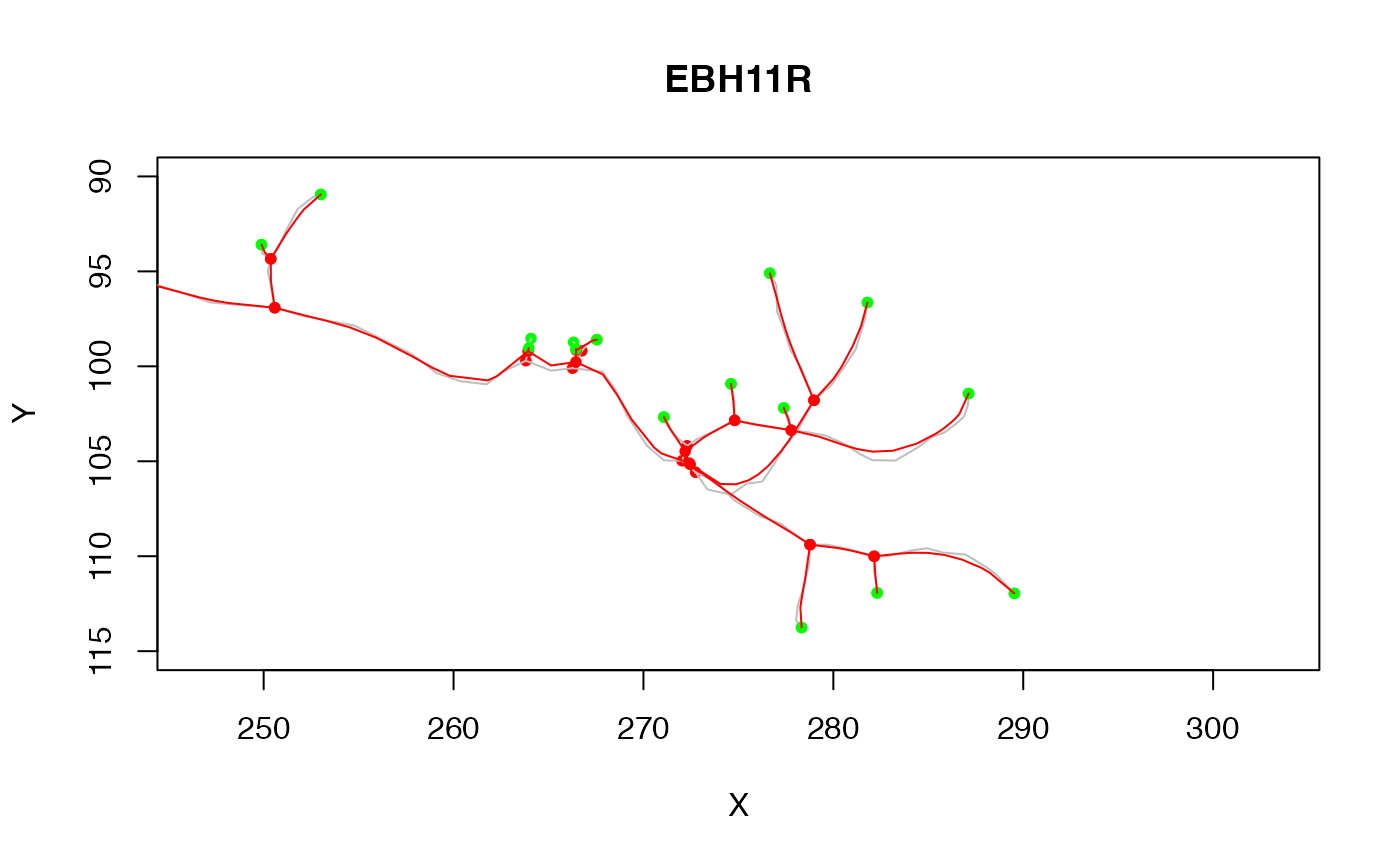smooth_neuron smooths a neuron.

smooth_neuron(n, method = c("gauss", "spline"), ...)

smooth_segment_gauss(xyz, sigma, ...)

## Arguments

n Neuron to smooth Smoothing method Additional parameters passed to segment smoothing functions A block of 3D coordinates defining an unbranched segment The standard deviation of the Gaussian smoothing kernel (which has the same spatial units as the object being smoothed)

## Value

A new neuron with smoothed 3d coordinates

## Examples

ns=smooth_neuron(Cell07PNs[], sigma=2)
# plot in 2D zooming in on axon terminals
plot(Cell07PNs[], col='grey', xlim=c(260,290), ylim=c(115,90))# \donttest{